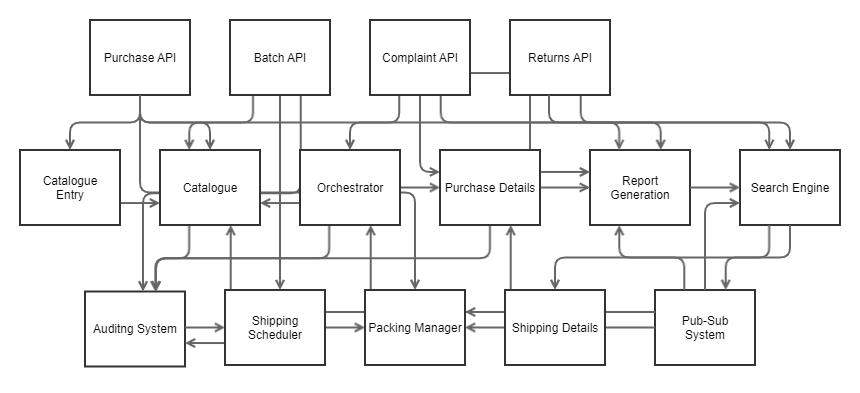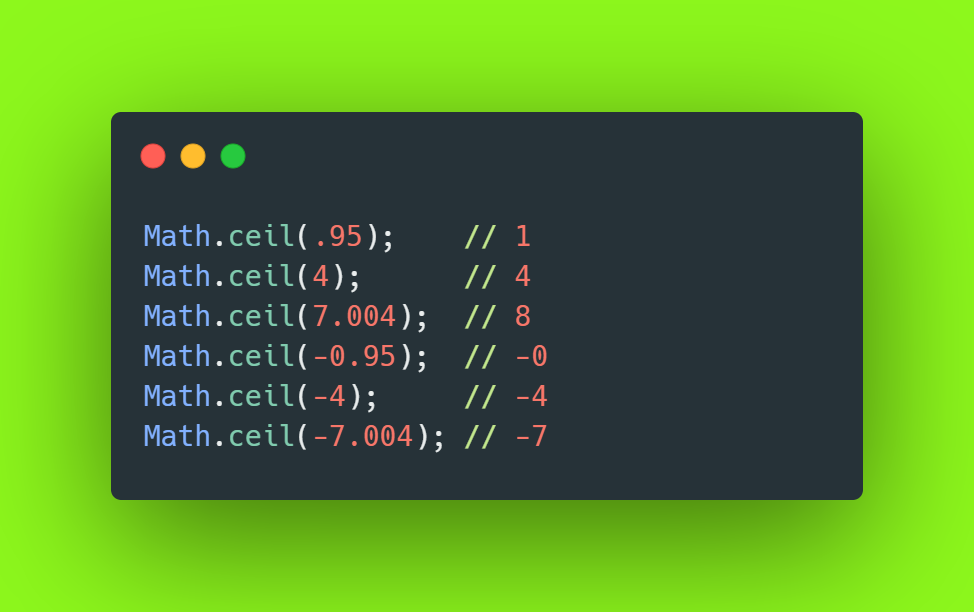1615520091

The Maths of Complexity

Why Microservices reduce the complexity of our software systems and how far we can push them.

We all know that creating big complicated monoliths is a bad idea, and creating microservices is the way to go, but why? Why are monoliths ‘bad’? Why are microservices ‘better’? Is there an optimum number of services that we should aim for when creating a system? This article takes a mathematical journey into complexity to uncover why microservices make sense, why monoliths are the great entropy monsters we think they are and what happens if we try to minimise complexity in our applications.

Microservices are a great way to structure software — they support Domain-Driven Design and allow us to align our deployment model with our delivered business value. Large monoliths, on the other hand, can be very complex, with a high degree of coupling between the parts. Sometimes we find it can be better to split them apart, creating subsystems, or, if we continue down this path – microservices. These smaller parts are easier to manage, fix and deploy. Practically speaking the smaller parts seem less complex overall and are less difficult to handle.

Let’s try to turn these imprecise statements into something more concrete. Is there a way in which we might compute the complexity of our applications, and can we see what happens to that complexity ‘value’ when we divide a monolithic application up into smaller parts?

The Maths Section

Let us start by considering a monolith which is comprised of 15 parts. These parts might be classes or components or services or files. Somehow or other we can describe the monolith as being comprised of these parts. The figure below shows a hypothetical monolith comprised of 15 subsystems. It’s not a real application so don’t pay too much attention to the details of the parts or their connections.#microservices #microservice architecture #software complexity

Buddha Community1624461000

MXReflection: A Java Math Framework

See what MXReflection can do for you.

**MXReflection **is a Java math framework based on mXparser library capabilities.

You can calculate complex mathematical operations and functions with Java, just by using class-related fields. MXReflection reads values from the assigned fields and injects the results in the @Expression annotated fields.

MXReflection Parsing

#java #framework #math #mxreflection #mxreflection: a java math framework #a java math framework1622622390

PhD Education - Hard PSLE Maths Questions

Solve any hard PSLE Maths questions with our comprehensive exam packages as we strive to help Primary 6 students in Singapore excel in upcoming exams.

#psle maths #hard psle maths questions #psle maths questions1620930540

Cognitive Complexity Vs Cyclomatic Complexity - An Example With C#a

Background

While writing professional code, code metrics are an important tool to determine that you are writing quality code that would make it easy to test, understand and maintain in the long run, as the code passes from one developer to another. Since every developer has a different style of writing the same logic, it becomes imperative to set up some standard/guidelines or quantitative measures to tell or determine what makes a good quality code. Two of the most relevant ones, that I find very interesting to explore is,

Cyclomatic Complexity and it’s younger sibling Cognitive Complexity!

The concept of Cognitive Complexity was brought in by SonarQube. They wanted to introduce a more contextualized form of measuring the code complexity. While you can read all the artifacts available to you in public domain on both this topic, I would rather summarize it as below, to the best of my understanding of the concepts till now,

Cyclomatic Complexity

Measures, how difficult it is test the code (i.e. Testability)._ Alternatively, this measure is a hint of how many distinct test cases you should write to have 100% code coverage. _

#c#a #cognitive complexity #cyclomatic complexity1599748140

Javascript Math Round Example | JS Math.round()

Javascript Math.round() is an inbuilt function that returns the value of a number rounded to the nearest integer. If the fractional portion of an argument is more than 0.5, the argument is turned to an integer with the next higher absolute value. If it is less than 0.5, then the argument is rounded to the integer with the lower value. If the fractional portion is exactly 0.5, then the argument is rounded to the next integer in the direction of +∞.

Javascript Math Round Example

The syntax of Math.round() is following.

Math.round(x)

Where x is a number. The value of a given number rounded to the nearest integer.

#javascript #math.round #js math.round1599754920

Math.ceil, Math.round and Math.floor in Your JavaScript

The universe is regulated by Math formulas” my grumpy teacher used to say. And the galaxy of coding isn’t escaping from that rule any time soon — as I was hoping. Luckily for us, a language like JavaScript gives us tools like the Math object to deal with numbers and their operations.

When it comes to rounding numbers, Math helps you with three main functions: Math.ceilMath.floorand Math.round.

Math.ceil

This method rounds up the only passed in value to the nearest greater integer. I find the word ceil meaningful in this case, as it reminds to a ceiling, to bringing something up. Or in our case, to raise a number to the next one.#technology #software-engineering #math #javascript #web-development